Mathematical and Physical Journal
for High Schools
Issued by the MATFUND Foundation
 Already signed up? New to KöMaL?

# KöMaL Problems in Physics, February 2016

Show/hide problems of signs:## Problems with sign 'M'

Deadline expired on March 10, 2016.

M. 357. Investigate the motion of a pencil, the cross section of which is a hexagon, and which was released from the top of an inclined plane from a horizontal position. Measure the average speed of the pencil, when the speed gets constant, as a function of the angle of elevation of the slope.

(6 pont)

solution, statistics## Problems with sign 'P'

Deadline expired on March 10, 2016.

P. 4805. A chain of length 30 m and of mass 40 kg is pulled up to a balcony at a height of 15, such that originally one end of the chain is already at the balcony. One half of the chain is hanging vertically and the other half lies on the ground in a small pile. How much work is done while the chain is pulled up?

(3 pont)

solution, statistics

P. 4806. A small piece of stone is thrown up vertically, which arrives back in 1.6 second. Find the maximal height of the stone and its initial velocity.

(3 pont)

solution, statistics

P. 4807. How many molecules are there in one litre of water, if the temperature of water is

$\displaystyle a)$ $\displaystyle 0~^\circ$C; $\displaystyle \,\,\,b)$ $\displaystyle 100~^\circ$C?

(3 pont)

solution, statistics

P. 4808. Luke and Sam are playing with a ball. First they throw the small ball vertically upwards, and they found that the greater the initial speed given the ball, the greater the height that it reaches.

Then they stand at some distance from each other and throw the ball to each other. Sam states that in this case it is also true that the greater the initial speed of the ball the higher it goes up. Luke doubts that this is always true.

Who is right?

(4 pont)

solution, statistics

P. 4809. The inhabitants of an Indian camp are shooting similar arrows into a wide wooden plank. Brainy Bear states that the red arrow penetrated twice as deeply into the plank as the blue arrow. He assumes that the resistive force exerted on the arrow is proportional to the depth of penetration. Thus he can answer to many different questions such as:

$\displaystyle a)$ By what factor was the speed of the red arrow at the impact greater than that of the blue one?

$\displaystyle b)$ Which arrow, the red or the blue one, had a longer braking time?

(4 pont)

solution, statistics

P. 4810. A body of mass 1 kg is suspended by means of a spring and a thread between two walls as shown in the figure. The measure of both angles between the thread and the horizontal and the spring and the horizontal is $\displaystyle 30^\circ$.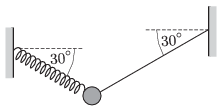$\displaystyle a)$ Determine the force exerted by the spring.

$\displaystyle b)$ What is the initial acceleration of the object if the thread breaks, and into which direction does the object begin to move?

(4 pont)

solution, statistics

P. 4811. A piston of mass $\displaystyle m=2~{\rm kg}$ confines an air column of height $\displaystyle \ell=30~{\rm cm}$ in a cylinder of cross section $\displaystyle A=10~{\rm cm}^2$. Both values of the internal and the external pressure are $\displaystyle p_0=10^5$ Pa. The cylinder can be rotated about a vertical axle. In the stationary position the middle of the piston is at a distance of $\displaystyle r=0.5$ m from the axle.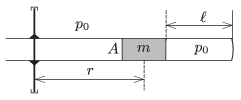$\displaystyle a)$ By what factor will the density of the gas increase if the cylinder is rotated with an angular speed of $\displaystyle \omega=3~{\rm s}^{-1}$.

$\displaystyle b)$ During the rotation, to what value should the external pressure be changed in order that the piston moves back to its original position? The temperature remains constant all the time.

(4 pont)

solution, statistics

P. 4812. There is a point-like light source $\displaystyle T$ on the principal axis of a converging lens at a distance of $\displaystyle d$ from the lens. There is a plane mirror on the other side of the lens opposite to the lens. The mirror is perpendicular to the principal axis.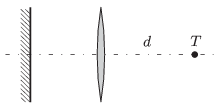$\displaystyle a)$ If the mirror is moved along the principal axis, then the image of the light source, formed by the system, always coincides with the light source. How can this happen?

$\displaystyle b)$ The distance between the light source and the lens is doubled. Where should the mirror be placed in order that parallel light rays leave the system.

(4 pont)

solution, statistics

P. 4813. Consider the bridge circuit shown in the figure.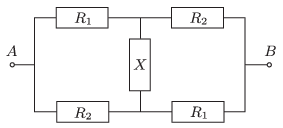$\displaystyle a)$ What is the resistance of the resistor in the middle if the equivalent resistance of the system between the points $\displaystyle A$ and $\displaystyle B$ is exactly the same as the resistance of the resistor in the middle: $\displaystyle R_{AB}=X$.

$\displaystyle b)$ Is it possible to choose the value of $\displaystyle X$ such that the equivalent resistance is equal to the root-mean-square value of $\displaystyle R_1$ and $\displaystyle R_2$, which is: $\displaystyle R_{AB}=\sqrt{\frac{R_1^2+R_2^2}{2}}$.

(4 pont)

solution, statistics

P. 4814. A thin uniform ring of mass $\displaystyle M$ has a charge $\displaystyle +Q$ distributed uniformly on it. It is at rest in empty space with no force acting on it. A particle of mass $\displaystyle m$ and charge $\displaystyle +q$ is projected towards the ring along its axis from infinity with a speed $\displaystyle v_0$, the minimum speed required by the particle to pass through the ring. The particle passes through the ring to move to an infinite distance from it in the opposite side.

$\displaystyle (i)$ What is the minimum speed of the particle during its entire motion?

$\displaystyle (ii)$ What is the maximum speed of the ring during the entire event?

$\displaystyle (iii)$ What are the final speeds of the particle and the ring at the end of the event?

[Neglect any loss of energy by the system due to radiation.]

(4 pont)

solution, statistics

P. 4815. Why is different the directionality of the loudspeakers for high and deep pitch? Accordingly, find an explanation of the fact that the deep sounds are intensified more, if the loudspeakers are placed directly in front of a wall.

(5 pont)

solution, statistics

P. 4816. A current of $\displaystyle I=25$ A flows in a piece of wide straight wire, which is fixed to a horizontal plane. The wire can be considered infinitely long. Next to the wire at a distance of $\displaystyle r_0=1$ cm from it there is a closed rectangle shaped loop of copper wire on the horizontal plane. The sides of the rectangle are $\displaystyle a=5$ cm, and $\displaystyle b=10$ cm. The current in the straight wire is decreased to zero uniformly in a very short time. What would the speed of the copper loop be if there was no frictional force exerted to it?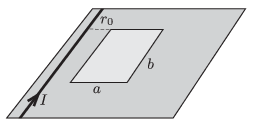(5 pont)

solution, statistics

P. 4817. The owner of a dog throws a ball into the river perpendicularly to the riverbank. The ball hits the water 5 metres away. The speed of the current is 0.3 m/s and the dog can swim at a speed of 0.5 m/s with respect to the water.

$\displaystyle a)$ Where and when will the dog reach the ball if it leaps into the water at the moment when the ball hit the water, and it swims towards the ball all the time?

$\displaystyle b)$ After reaching the ball the dog immediately starts to swim towards its owner. The motion of the dog is uniform straight line motion, such that the direction of the velocity of the dog with respect to the riverbank points towards the owner all the time. How much time elapses from the moment the dog reached the ball until it gives it back to its owner?

$\displaystyle c)$ The owner throws the ball again into the water in the same way as he did previously. The dog reaches the ball again, but when it brings it back it swims along a curved path such that its velocity with respect to the water points towards its owner. After reaching the ball how much time elapses until the dog gives the ball back to its owner? (Hint: Compare the rate of change of the distance between the owner and the dog to that component of the dog's velocity, which is parallel to the current.)

In which case is the time during which the dog brings back the ball shorter, and what is the time difference between these two cases?

(6 pont)

solution, statistics